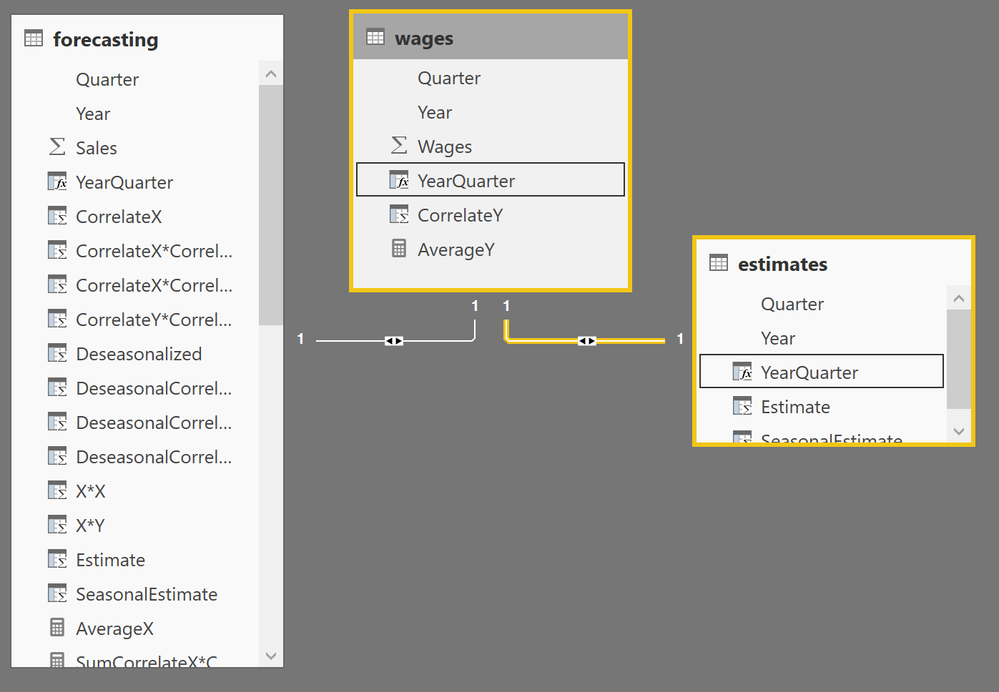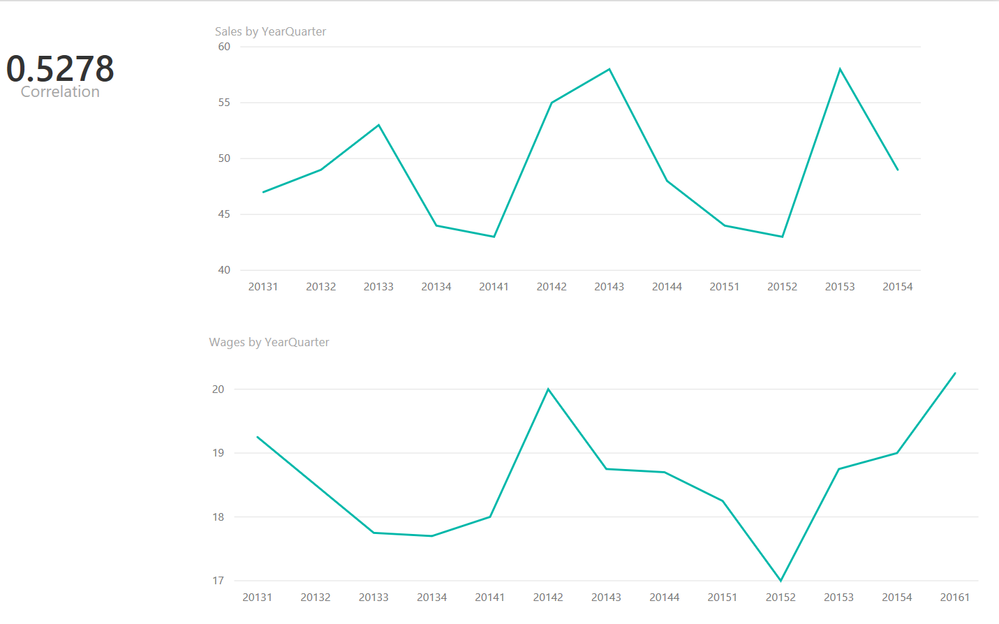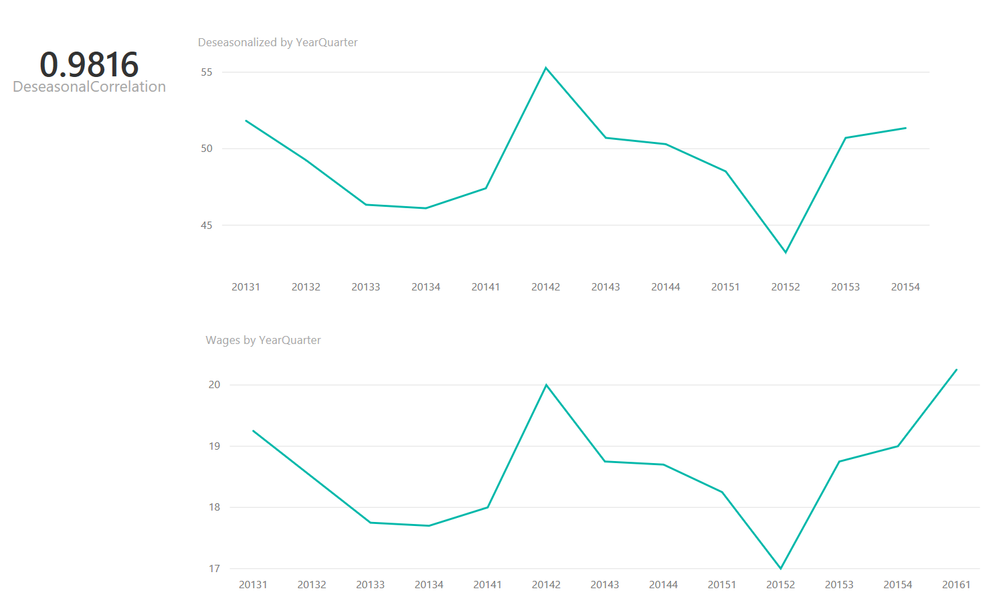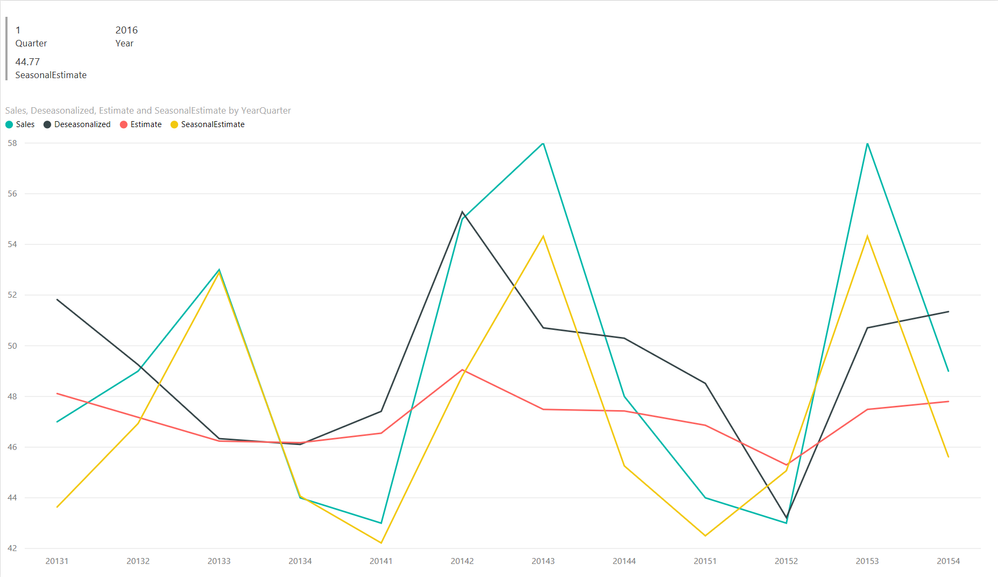cancel
Showing results for
Did you mean:## Correlation, Seasonality and Forecasting with Power BI

Scenario

A retail firm believes that its sales are driven by an external index that we will call the "Real Wage Index". This index tracks real hourly wages adjusted for inflation, seasonality, etc. The retail firm believes that this index is a 3-month leading indicator, meaning that when the index goes up, the retail store sees its sales go up 3 months later and when the index goes down, the store sales go down 3 months later.

Method

Starting with the data, the company has the following sales data from the past three years. Sales are in millions of US dollars.

forecasting.csv

Quarter,Year,Sales

1,2013,47

2,2013,49

3,2013,53

4,2013,44

1,2014,43

2,2014,55

3,2014,58

4,2014,48

1,2015,44

2,2015,43

3,2015,58

4,2015,49

The real wage index for roughly the same time period is:

wages.csv

Quarter,Year,Wages

2,2013,19.25

3,2013,18.50

4,2013,17.75

1,2014,17.70

2,2014,18.00

3,2014,20.00

4,2014,18.75

1,2015,18.70

2,2015,18.25

3,2015,17.00

4,2015,18.75

1,2016,19.00

2,2016,20.25

Finally, the retail firm is attempting to estimate sales for the first quarter of 2016:

estimates.csv

Quarter,Year

1,2016

To start the analysis, these three files are loaded into Power BI Desktop as forecasting, wages and estimates tables. Once the tables are loaded, we set the Quarter and Year columns to "Do not summarize" in the model.

Since we believe that the wage index and the firm's sales are related, we want to create a relationship between the two tables, wages and forecasting respectively. To do this, we need a single key. In the forecasting table, we create a new column with the following formula:

YearQuarter = [Year] & [Quarter]

In the wages table, we create a similar column but we must adjust it to reflect our hypothesized 3-month leading indicator status.

YearQuarter = IF([Quarter] = 1,[Year]-1 & "4",[Year] & [Quarter]-1)

Essentially, this formula offsets the quarter by one so that when we relate the two tables by YearQuarter, we have adjusted for the 3-month lead in the related wage index.

Finally, in the estimates table, we create a new column with the following formula:

YearQuarter = [Year] & [Quarter]

We then create relationships between forecasting and wages using the YearQuarter columns in those tables as well as a relationship between estimates and wages using the YearQuarter columns. Once complete, the relationships in our model should look like the following:Our next task is to see if the two series of data are, in fact, related to one another. We can do this via Pearson's Correlation. While Excel has the CORREL function, we can also do this in Power BI Desktop (and use Excel to verify our result). When using Pearson's Correlation, the calculated correlation falls between 1 and -1, inclusive. A result of 1 is a perfect positive correlation. A result of 0 is no correlation and a value of -1 is perfect negative correlation. Values between these numbers indicate the strength of the correlation. For example, a value of .5 would be low positive correlation while a value of .9 would be high positive correlation. Positive correlation means that the when one value increases, the related value increases and vice versa. Negative correlation means that the two series are inversly related. For example, when one value decreases, the related value increases and vice versa.

To do this in Power BI Desktop, we arbitrarily assign the "x" variable to Sales and the "y" value to wages and then take the following steps:

• In forecasting, create new measure: AverageX = AVERAGEX(ALL(forecasting),[Sales])
• In wages, create new measure: AverageY = AVERAGEX(ALL(wages),[Wages])
• In forecasting, create new column: CorrelateX = [Sales] - [AverageX]
• In wages, create new column: CorrelateY = [Wages] - [AverageY]
• In forecasting create new column: CorrelateX*CorrelateX = [CorrelateX] * [CorrelateX]
• In forecasting create new column: CorrelateX*CorrelateY = [CorrelateX] * RELATED(wages[CorrelateY])
• In forecasting create new column: CorrelateY*CorrelateY = RELATED(wages[CorrelateY]) * RELATED(wages[CorrelateY])
• In forecasting, create new measure: SumCorrelateX*CorrelateX = SUMX(ALL(forecasting),[CorrelateX*CorrelateX])
• In forecasting, create new measure: SumCorrelateX*CorrelateY = SUMX(ALL(forecasting),[CorrelateX*CorrelateY])
• In forecasting, create new measure: SumCorrelateY*CorrelateY = SUMX(ALL(forecasting),[CorrelateY*CorrelateY])
• In forecasting, create new measure: Correlation = [SumCorrelateX*CorrelateY] / SQRT([SumCorrelateX*CorrelateX]*[SumCorrelateY*CorrelateY])

Once we have the new columns and measures, we can create a report that shows our Correlation measure and we can also plot Wages and Sales figures by YearQuarter to visually see their potential correlation.Unfortunately, we find that our resulting correlation calculation (Correlation measure) is not particularly strong, a mere .5278. This is also evident in the graphs of the figures by YearQuarter. However, it is often the case with time series data that data such as sales numbers are impacted by seasonality. Seasonality is essentially a pattern of demand that repeats at a particular time interval. Seasonality might be yearly, monthly, weekly or even daily. The issue with seasonality is that it artifiially skews the numbers based upon some reoccurring, time sensitive event. Because of this, forecasting techniques such as linear regression and exponential smoothing do not do a good job when seasonality is present. In addition, if one is trying to find a correlation between two data series that so not have the same seasonality, calculated correlation values can be found to be weaker than they actually are. The solution to seasonality is to deseasonalize the data.

Given the retail nature of the business, it is reasonable to assume annual seasonality is present. Therefore, we can deseasonalize the sales data by following these steps:

• In forecasting, create new measure: SeasonQuarter1Average = CALCULATE(AVERAGE([Sales]),forecasting[Quarter] = 1)
• In forecasting, create new measure: SeasonQuarter2Average = CALCULATE(AVERAGE([Sales]),forecasting[Quarter] = 2)
• In forecasting, create new measure: SeasonQuarter3Average = CALCULATE(AVERAGE([Sales]),forecasting[Quarter] = 3)
• In forecasting, create new measure: SeasonQuarter4Average = CALCULATE(AVERAGE([Sales]),forecasting[Quarter] = 4)
• In forecasting, create new measure: SeasonAverage = AVERAGEX(ALL(forecasting),[Sales])
• In forecasting, create new measure: SeasonQ1SI = CALCULATE([SeasonQuarter1Average]/[SeasonAverage],ALL(forecasting))
• In forecasting, create new measure: SeasonQ2SI = CALCULATE([SeasonQuarter2Average]/[SeasonAverage],ALL(forecasting))
• In forecasting, create new measure: SeasonQ3SI = CALCULATE([SeasonQuarter3Average]/[SeasonAverage],ALL(forecasting))
• In forecasting, create new measure: SeasonQ4SI = CALCULATE([SeasonQuarter4Average]/[SeasonAverage],ALL(forecasting))
• In forecasting, create new column: Deseasonalized = IF([Quarter]=1,[Sales]/[SeasonQ1SI],IF([Quarter]=2,[Sales]/[SeasonQ2SI],IF([Quarter]=3,[Sales]/[SeasonQ3SI],[Sales]/[SeasonQ4SI])))

We can now recalculate the correlation using the deseasonalized sales figures. To do this, perform these steps:

• In forecasting, create new measure: DeseasonalAverageX = AVERAGEX(ALL(forecasting),[Deseasonalized])
• In forecasting, create new column: DeasonalCorrelateX = [Deseasonalized] - [DeseasonalAverageX]
• In forecasting, create new column: DeseasonalCorrelateX*DeseasonalCorrelateX = [DeseasonalCorrelateX] * [DeseasonalCorrelateX]
• In forecasting, create new column: DeseasonalCorrelateX*CorrelateY = [DeseasonalCorrelateX] * RELATED(wages[CorrelateY])
• In forecasting, create new measure: SumDeseasonalCorrelateX*DeseasonalCorrelateX = SUMX(ALL(forecasting),[DeseasonalCorrelateX*DeseasonalCorrelateX])
• In forecasting, create new measure: SumDeseasonalCorrelateX*CorrelateY = SUMX(ALL(forecasting),[DeseasonalCorrelateX*CorrelateY])
• In forecasting, create new measure: DeseasonalCorrelation = [SumDeseasonalCorrelateX*CorrelateY] / SQRT([SumDeseasonalCorrelateX*DeseasonalCorrelateX]*[SumCorrelateY*CorrelateY])

We can create another report to see the correlation by deseasonalized sales numbers.We can now see that the DeseasonalCorrelation measure is .9816, indicating a very strong correlation between the wage index as a 3-month leading indicator and retail sales. This means that we can use the wage index as a predictive measure to forecast sales. To do this, we will use linear regression. Linear regression is a mathematical method used to find the "best fit" of a straight line through a series of data points. There are a number of different linear regression methods, the one that we will use is known as "simple linear regression". For more information on simple linear regression, see the following link: https://en.wikipedia.org/wiki/Simple_linear_regression.

While previously we arbitrarily assigned our variables as "x" and "y", we will now follow the convention of calling our independent variable (Wages) "x" and our dependent variable (Sales) "y".

• In forecasting, create new column: X*X = RELATED(wages[Wages]) * RELATED(wages[Wages])
• In forecasting, create new column: X*Y = RELATED(wages[Wages]) * [Deseasonalized]
• In forecasting, create new measure: SumX = SUMX(ALL('wages'),[Wages])
• In forecasting, create new measure: SumY = SUMX(ALL('forecasting'),[Deseasonalized])
• In forecasting, create new measure: SumX*X = SUMX(ALL('forecasting'),[X*X])
• In forecasting, create new measure: SumX*Y = SUMX(ALL('forecasting'),[X*Y])
• In forecasting, create new measure: Slope = ([Count]*[SumX*Y] - [SumX]*[SumY]) / ([Count]*[SumX*X] - [SumX]*[SumX])
• In forecasting, create new measure: Intercept = ([SumY] - [Slope]*[SumX]) / [Count]
• In forecasting, create new column: Estimate = [Intercept] + [Slope]*RELATED(wages[Wages])
• In estimates, create new column: Estimate = [Intercept] + [Slope]*RELATED(wages[Wages])

This process curve fits a straight line through the deseasonalized data. However, in order to get our true estimates of sales, we need to reseasonalize the sales estimates. To do this, we perform the following steps:

• In forecasting, create new column: SeasonalEstimate = IF([Quarter]=1,[Estimate]*[SeasonQ1SI],IF([Quarter]=2,[Estimate]*[SeasonQ2SI],IF([Quarter]=3,[Estimate]*[SeasonQ3SI],[Estimate]*[SeasonQ4SI])))
• In estimates, create new column: SeasonalEstimate = IF([Quarter]=1,[Estimate]*[SeasonQ1SI],IF([Quarter]=2,[Estimate]*[SeasonQ2SI],IF([Quarter]=3,[Estimate]*[SeasonQ3SI],[Estimate]*[SeasonQ4SI])))

Once this is complete, we can create a final report that displays our reseasonalized estimate for the first quarter of 2016, 44.77 as well as a graph that displays Sales, Deseasonalized Sales (Deseasonlized), Deseasonlized Estimate (Estimate) and Reseasonalized Estimate (SeasonalEstimate) in order to visually see their relationships.Conclusion

It is possible in Power BI to correlate two time series data sets, remove seasonality and do forecasting.